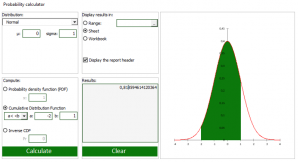# Probability Calculator

Use this tool to compute for a given distribution function, the density function, the cumulative distribution function, or the inverse cumulative distribution function. Available in Excel with the XLSTAT software.## What is a probability distribution?

A probability distribution is a special type of function, that is named a measure in mathematics. It allows to relate events (for example, the occurrence of a 2 when throwing a die), to their probability.

A large number of probability distributions have been developed to describe particular situations where randomness occurs. Randomness is a representation of ignorance or imperfect knowledge. When you roll a die, a perfect knowledge of the starting movement allow you knowing when, where, and in what position the die would stop. Nevertheless, it is so complex that it is preferable to estimate that each face has a certain probability of being the result of the throw and that the occurrence of an event is random.

A random variable is also a function that maps an event to a real. For example, we can match the tossing of a coin with 0 if the coin lands on head, and 1 if the coin lands on tail. While this is perfectly arbitrary in the case of a coin, it may be more natural if one counts the number of people in a queue at the post office, or if one measures the air temperature. In this case, we will match the event "there are 10 people queuing" with the number 10, or "the temperature is 59.8°F" with the real 59.8.

In the case of discrete variables, each event has a non-zero probability as long as it is possible. In the case of continuous variables, each (possible) event has a non-zero probability of occurring, but it is so small that only the probability distribution makes it possible to measure it. While the measure does not mean much by itself, it allows to compare the relative chances to occur of two events. It also allows, through a sum (an integral) to give the probability of a series of events (described by an interval) to occur. Thus, for example, if a measurement of the temperature is made and the measurement is known to be error-prone and if we assume the measurement follows a normal distribution (the famous distribution with a bell shape), the event "it is 60°F", has virtually a null probability to occur, while on the other hand we will be able to give the probability that we measure a temperature between 59.5°F and 60.5°F. The mathematical tool for calculating this probability is called the cumulative distribution function (CDF).

Two other functions are commonly used:

• The probability density function: This function is the function to integrate to calculate the cumulative distribution function, which is valid for the case of variables with density (which is true for all distributions proposed by XLSTAT).
• The inverse cumulative distribution function: This function allows, for a given probability to obtain the value x of the random variable such that the distribution function in x is p.

## Probability Calculator in XLSTAT

The probability calculator allows to calculate, for all distributions proposed by XLSTAT, the probability density function, the cumulative distribution function and the inverse cumulative distribution function.

The results displayed correspond to the probability distribution function and to the value computed for the selected function (CDF, PDF or inverse CDF) at a specific point.### analysieren sie ihre daten mit xlstat

kostenlose 14-tage-testversion

Enthalten ind

Verwandte Funktionen